# 使用 MATLAB 和 Dcraw 处理 RAW 图像文件

## Dcraw 预处理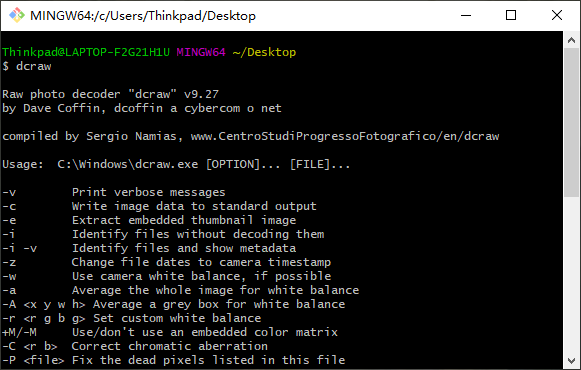1  dcraw -4 -T -D -v pathfilename 

 1  dcraw -4 -T -D -v image.CR2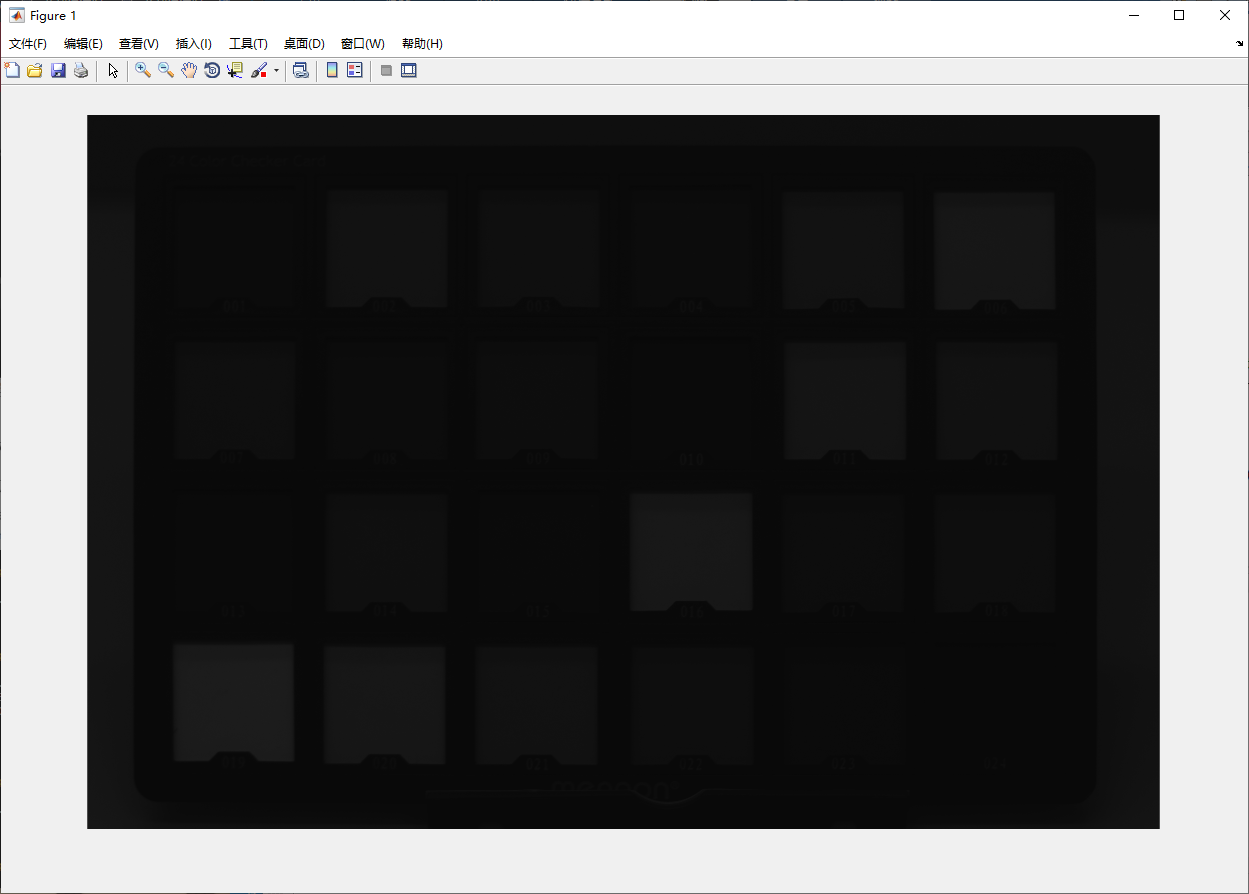1  raw = double(imread('image.tiff')); 

## 线性处理

 1 2 3 4  black = 2047; saturation = 13584; lin_bayer = (raw-black)/(saturation-black); % 归一化至 [0,1] lin_bayer = max(0,min(lin_bayer,1)); % 确保没有大于 1 或小于 0 的数据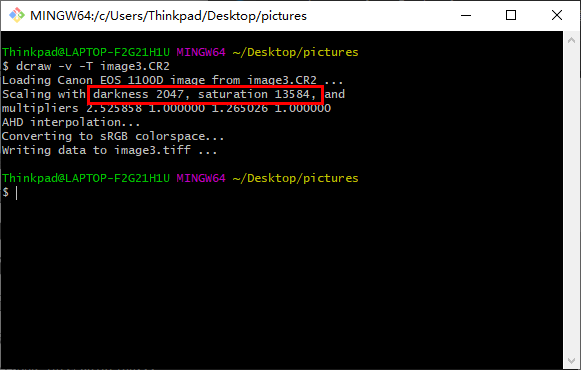## 白平衡校正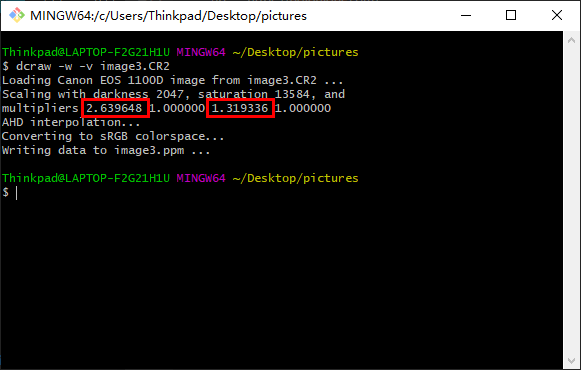1 2 3  wb_multipliers = [2.639648, 1, 1.319336]; % for particular condition, from dcraw; mask = wbmask(size(lin_bayer,1),size(lin_bayer,2),wb_multipliers,'rggb'); balanced_bayer = lin_bayer .* mask; 

  1 2 3 4 5 6 7 8 9 10 11 12 13 14 15 16 17 18 19 20 21  function colormask = wbmask(m,n,wbmults,align) % COLORMASK = wbmask(M,N,WBMULTS,ALIGN) % Makes a white-balance multiplicative mask for an image of size m-by-n % with RGB while balance multipliers WBMULTS = [R_scale G_scale B_scale]. % ALIGN is string indicating Bayer arrangement: 'rggb','gbrg','grbg','bggr' colormask = wbmults(2) * ones(m,n); % Initialize to all green values; switch align case 'rggb' colormask(1:2:end,1:2:end) = wbmults(1); colormask(2:2:end,2:2:end) = wbmults(3); case 'bggr' colormask(2:2:end,2:2:end) = wbmults(1); colormask(1:2:end,1:2:end) = wbmults(3); case 'grbg' colormask(1:2:end,2:2:end) = wbmults(1); colormask(2:2:end,1:2:end) = wbmults(3); case 'gbrg' colormask(2:2:end,1:2:end) = wbmults(1); colormask(1:2:end,2:2:end) = wbmults(3); end end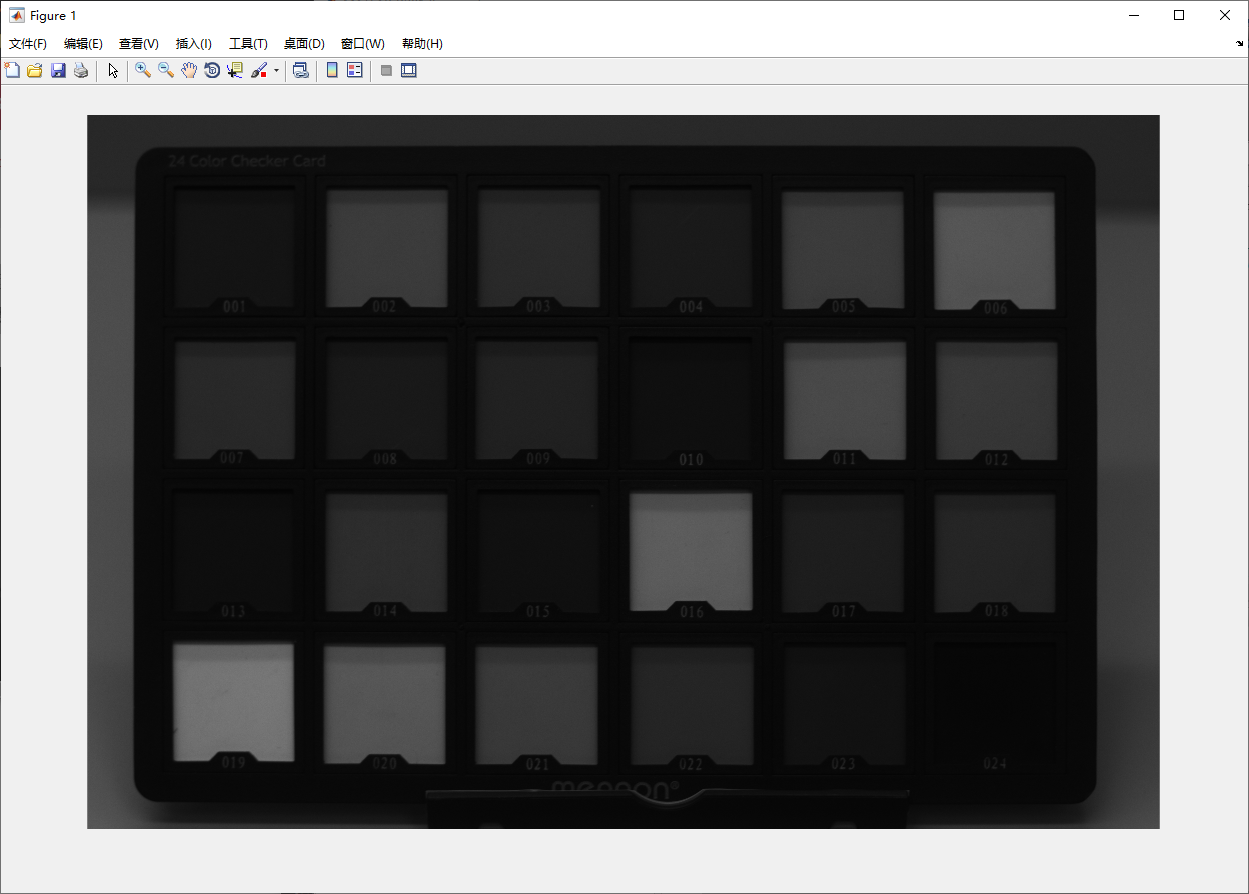## 色彩差值

 1 2  temp = uint16(balanced_bayer/max(balanced_bayer(:)) * (2^16-1)); lin_rgb = double(demosaic(temp,'rggb'))/(2^16-1);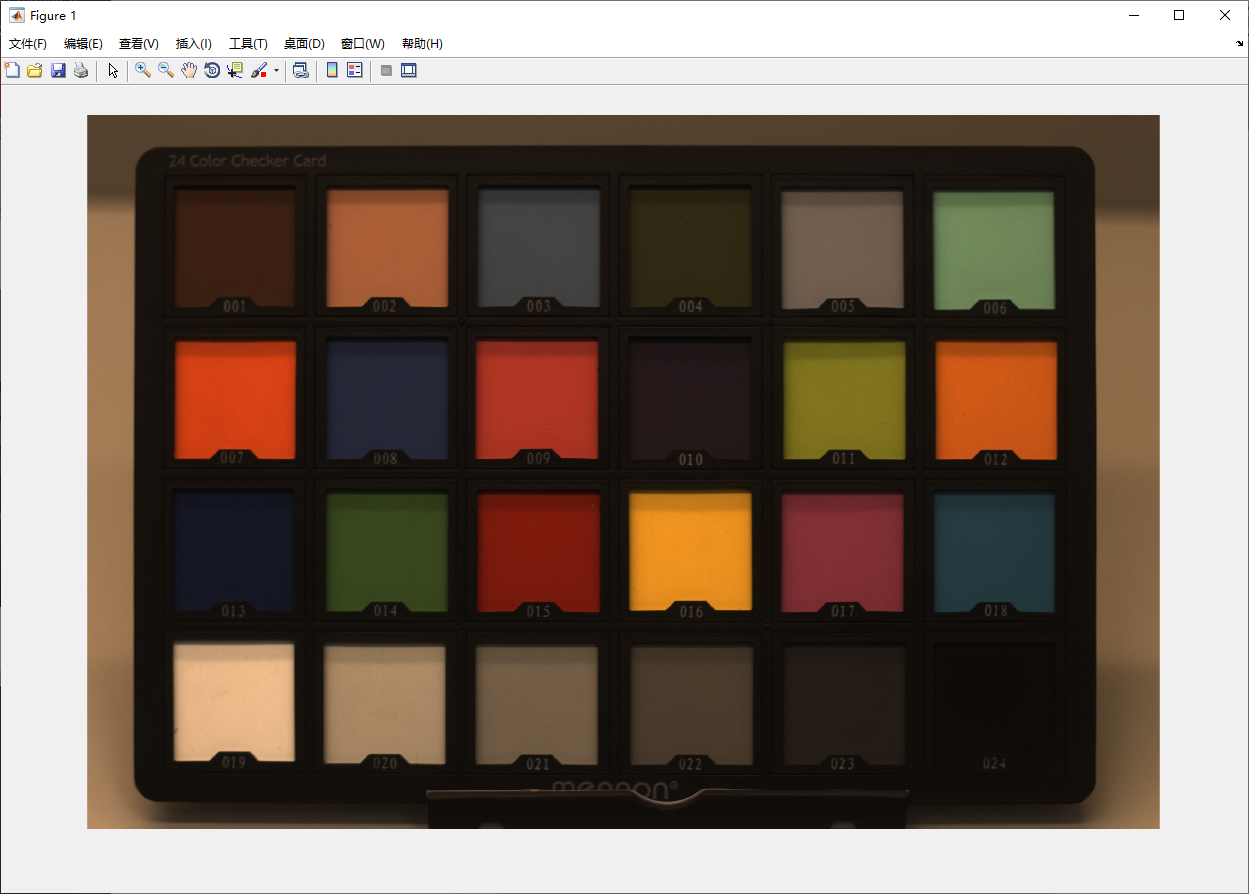## 色彩空间转换

$$A_{sRGB\leftarrow Camera}=(A_{Camera\leftarrow XYZ}\cdot A_{XYZ\leftarrow sRGB})^{-1}$$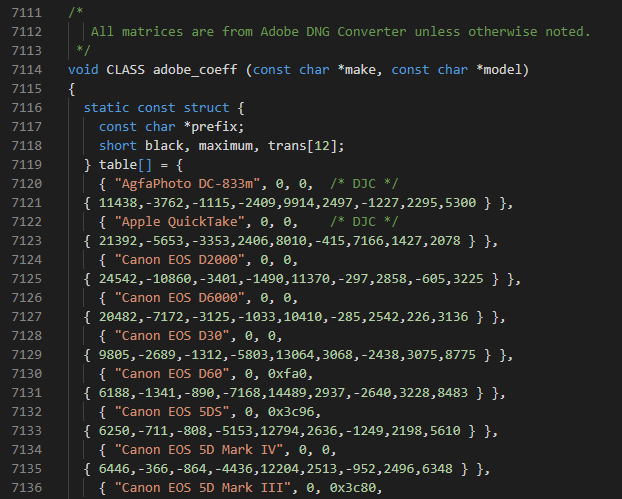1 2  { "Canon EOS 1100D", 0, 0x3510, { 6444,-904,-893,-4563,12308,2535,-903,2016,6728 } } 

$$A_{Camera\leftarrow XYZ}=\frac{1}{10000} \begin{bmatrix} 6444&-904&-893\\ -4563&12308&2535\\ -903&2016&6728 \end{bmatrix}$$

sRGB-to-XYZ 可以在国际照明委员会（CIE）公布的标准中查到，有：

$$A_{XYZ\leftarrow sRGB}= \begin{bmatrix} 0.4124564&0.3575761&0.1804375\\ 0.2126729&0.7151522&0.0721750\\ 0.0193339&0.1191920&0.9503041 \end{bmatrix}$$

$$\begin{bmatrix} 1\\ 1\\ 1 \end{bmatrix}_{Camera} = \Bigg[A_{Camera\leftarrow sRGB}\Bigg] \begin{bmatrix} 1\\ 1\\ 1 \end{bmatrix}_{sRGB}$$

 1 2 3 4 5 6 7 8 9  sRGB2XYZ = [0.4124564 0.3575761 0.1804375;0.2126729 0.7151522 0.0721750;0.0193339 0.1191920 0.9503041]; % sRGB2XYZ is an unchanged standard XYZ2Cam = [6444,-904,-893;-4563,12308,2535;-903,2016,6728]/10000; % Here XYZ2Cam is only for Canon EOS 1100D, can be found in adobe_coeff in dcraw.c sRGB2Cam = XYZ2Cam * sRGB2XYZ; sRGB2Cam = sRGB2Cam./ repmat(sum(sRGB2Cam,2),1,3); % normalize each rows of sRGB2Cam to 1 Cam2sRGB = (sRGB2Cam)^-1; lin_srgb = apply_cmatrix(lin_rgb, Cam2sRGB); lin_srgb = max(0,min(lin_srgb,1)); % Always keep image clipped b/w 0-1 

  1 2 3 4 5 6 7 8 9 10 11  function corrected = apply_cmatrix(im,cmatrix) % Applies CMATRIX to RGB input IM. Finds the appropriate weighting of the % old color planes to form the new color planes, equivalent to but much % more efficient than applying a matrix transformation to each pixel. if size(im,3) ~=3 error('Apply cmatrix to RGB image only.'); end r = cmatrix(1,1) * im(:,:,1)+cmatrix(1,2) * im(:,:,2)+cmatrix(1,3) * im(:,:,3); g = cmatrix(2,1) * im(:,:,1)+cmatrix(2,2) * im(:,:,2)+cmatrix(2,3) * im(:,:,3); b = cmatrix(3,1) * im(:,:,1)+cmatrix(3,2) * im(:,:,2)+cmatrix(3,3) * im(:,:,3); corrected = cat(3,r,g,b);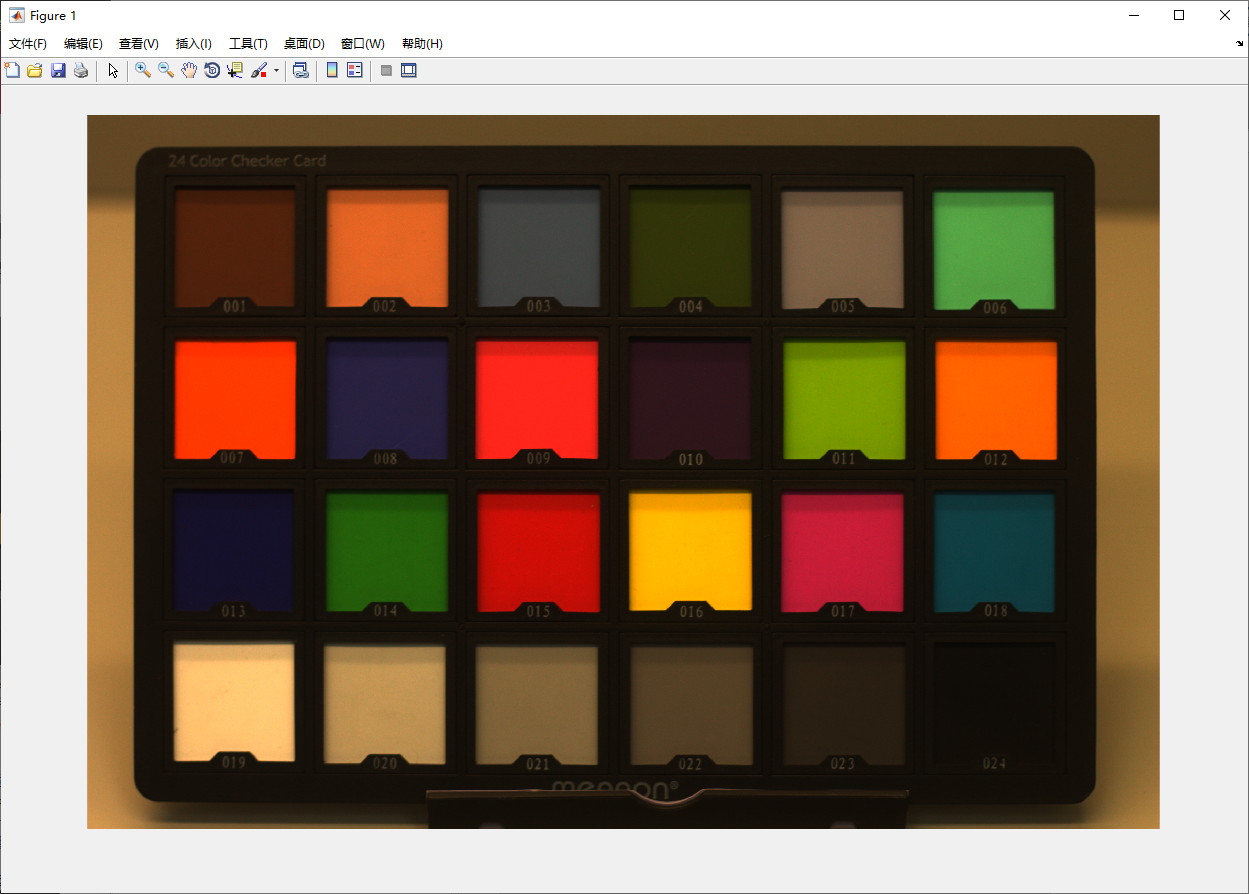## 亮度校正与伽马校正

 1 2 3  grayim = rgb2gray(lin_srgb); % Consider only gray channel grayscale = 0.25/mean(grayim(:)); bright_srgb = min(1,lin_srgb * grayscale); % Always keep image value less than 1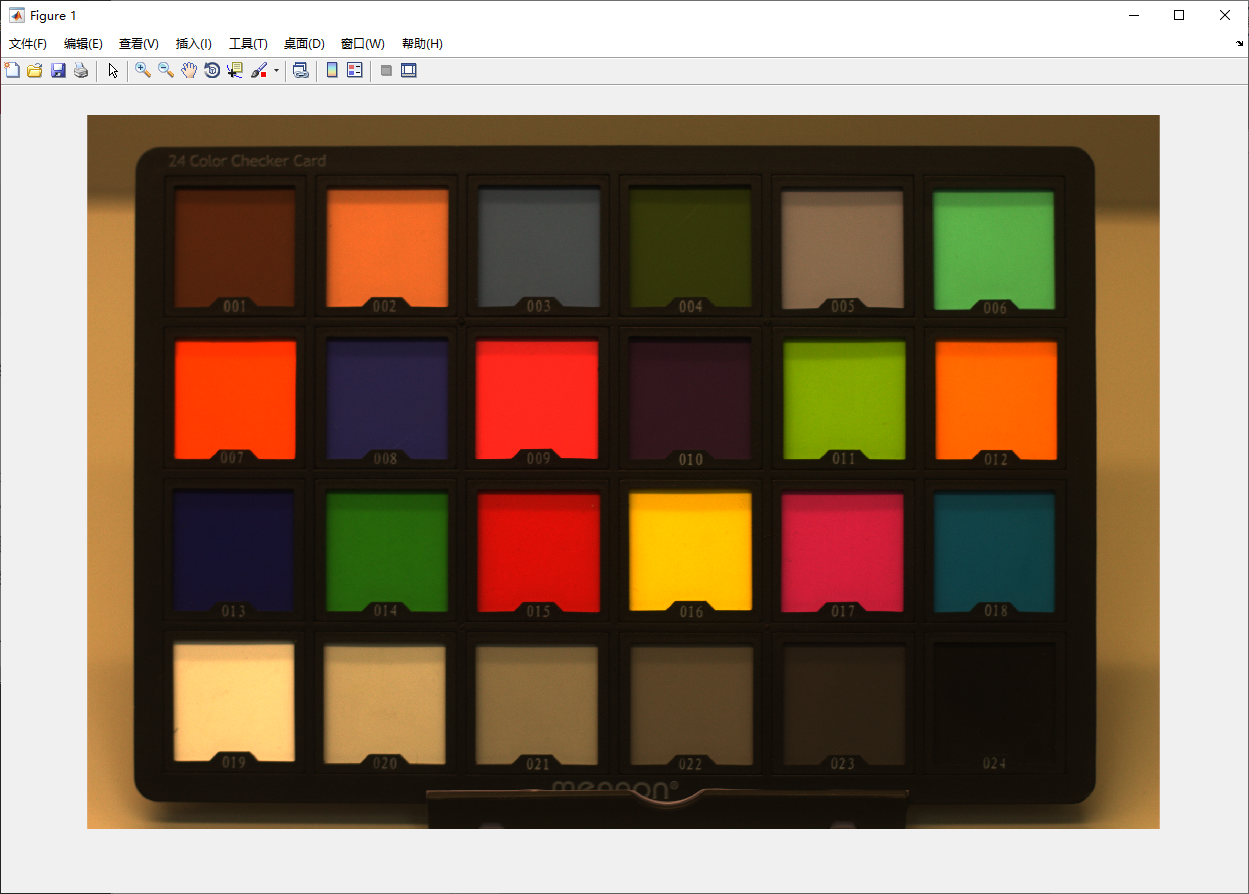1  nl_srgb = bright_srgb.^(1/2.2);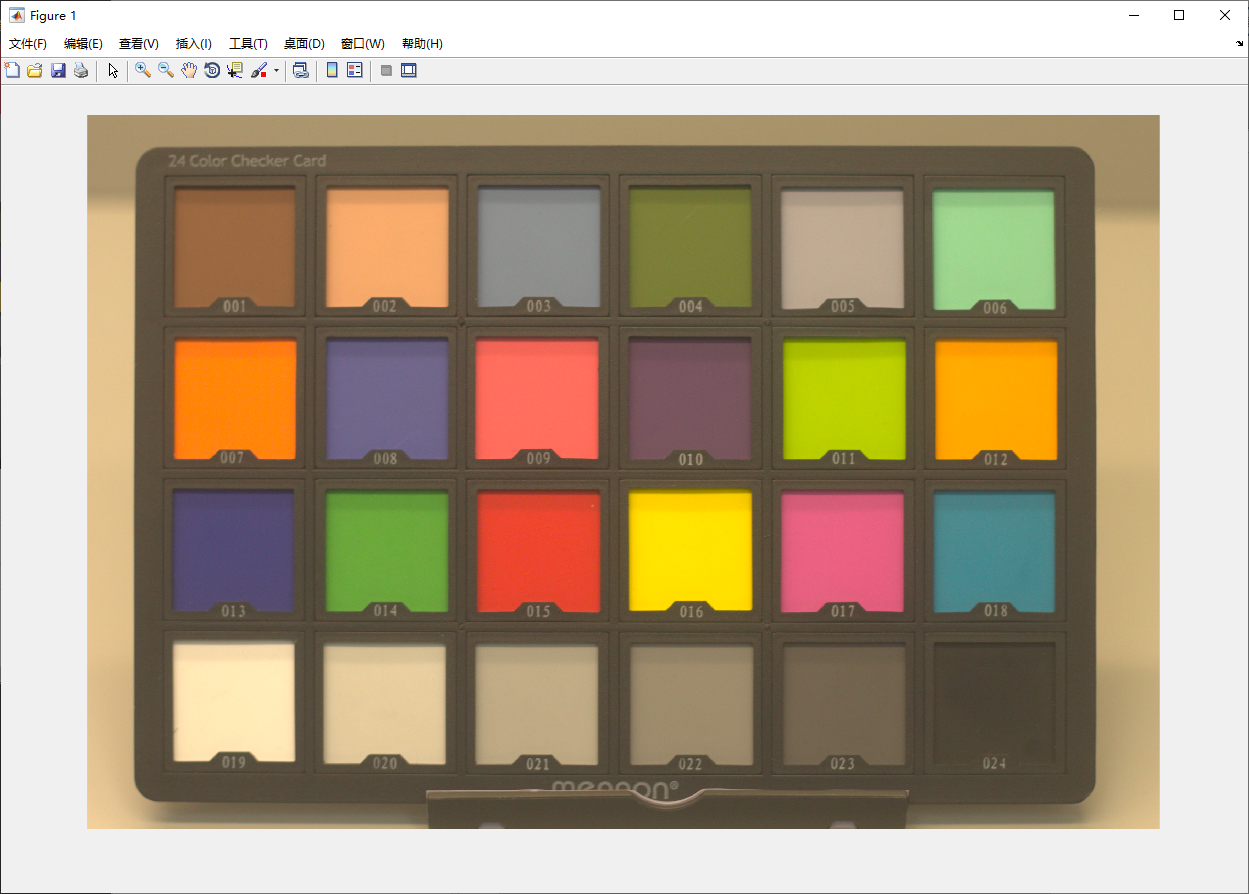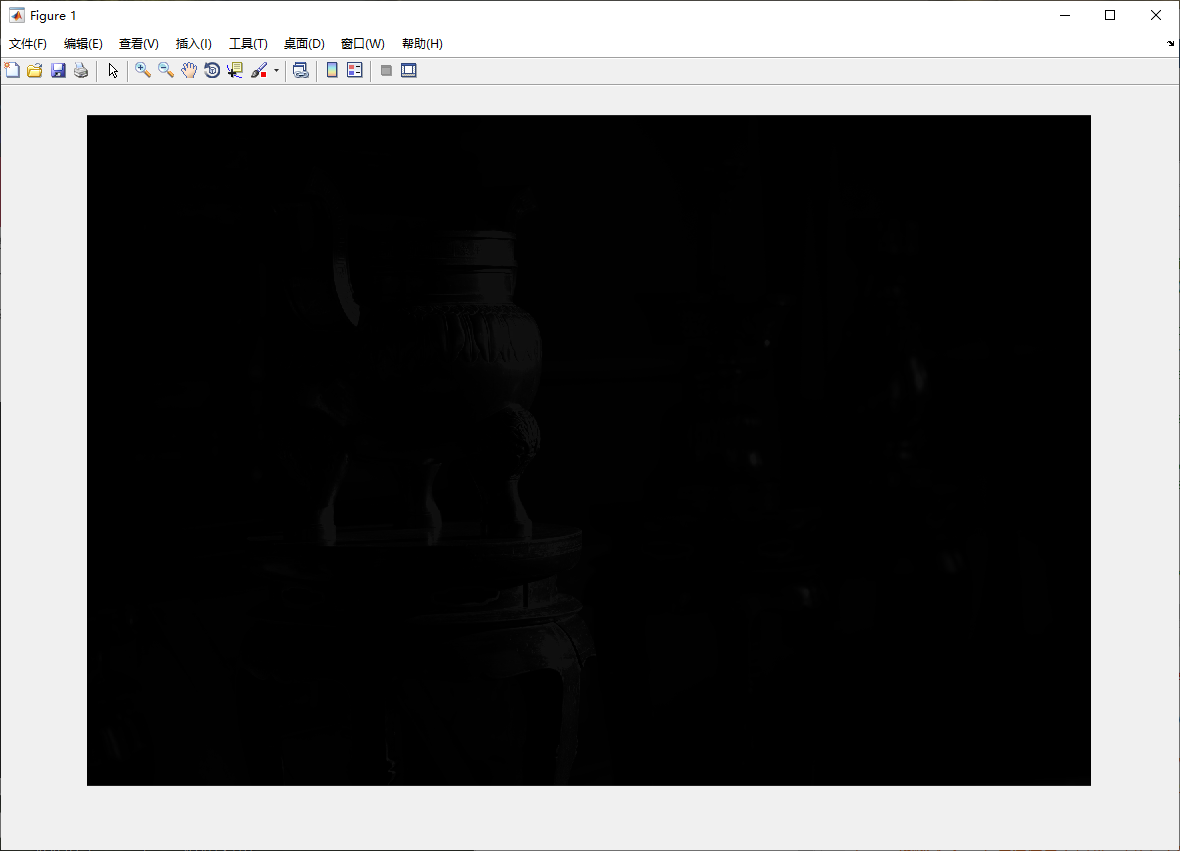◎ 直接读取的 tiff 文件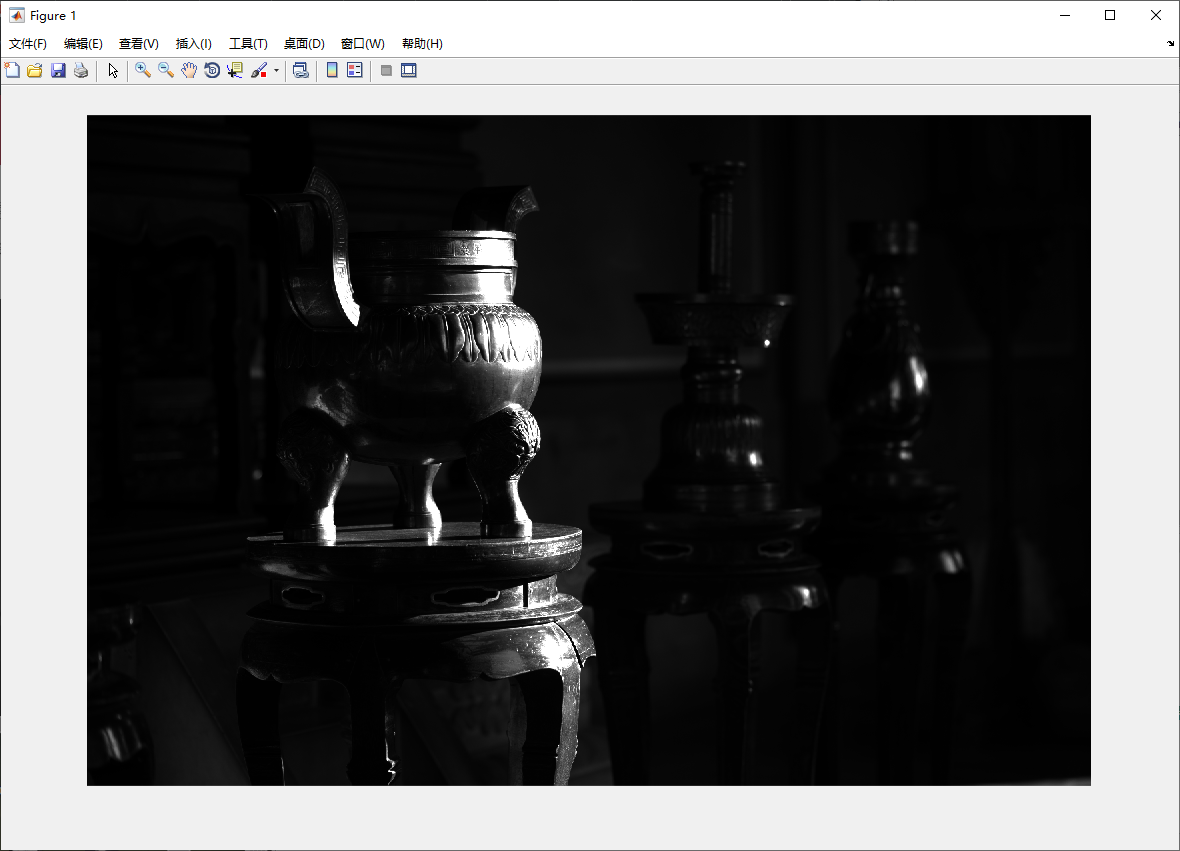◎ 线性处理后的图像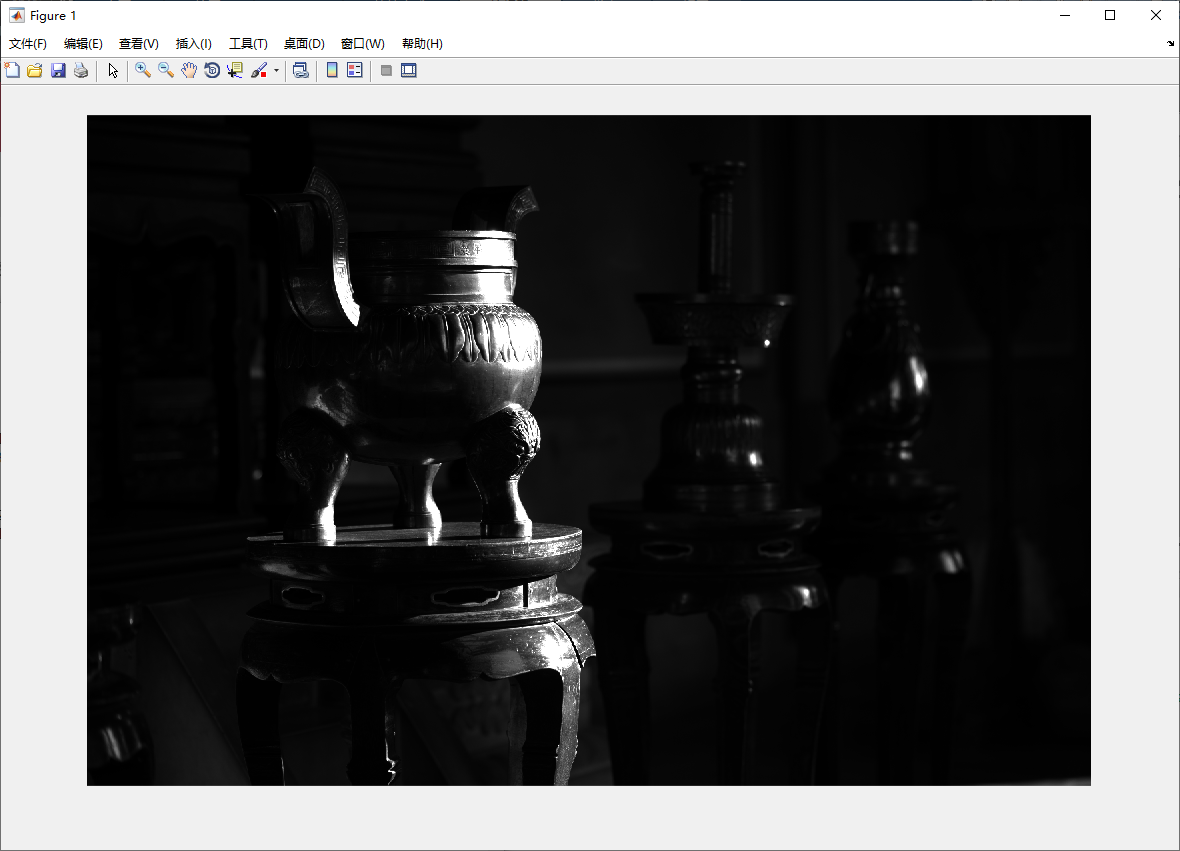◎ 白平衡调整后的图像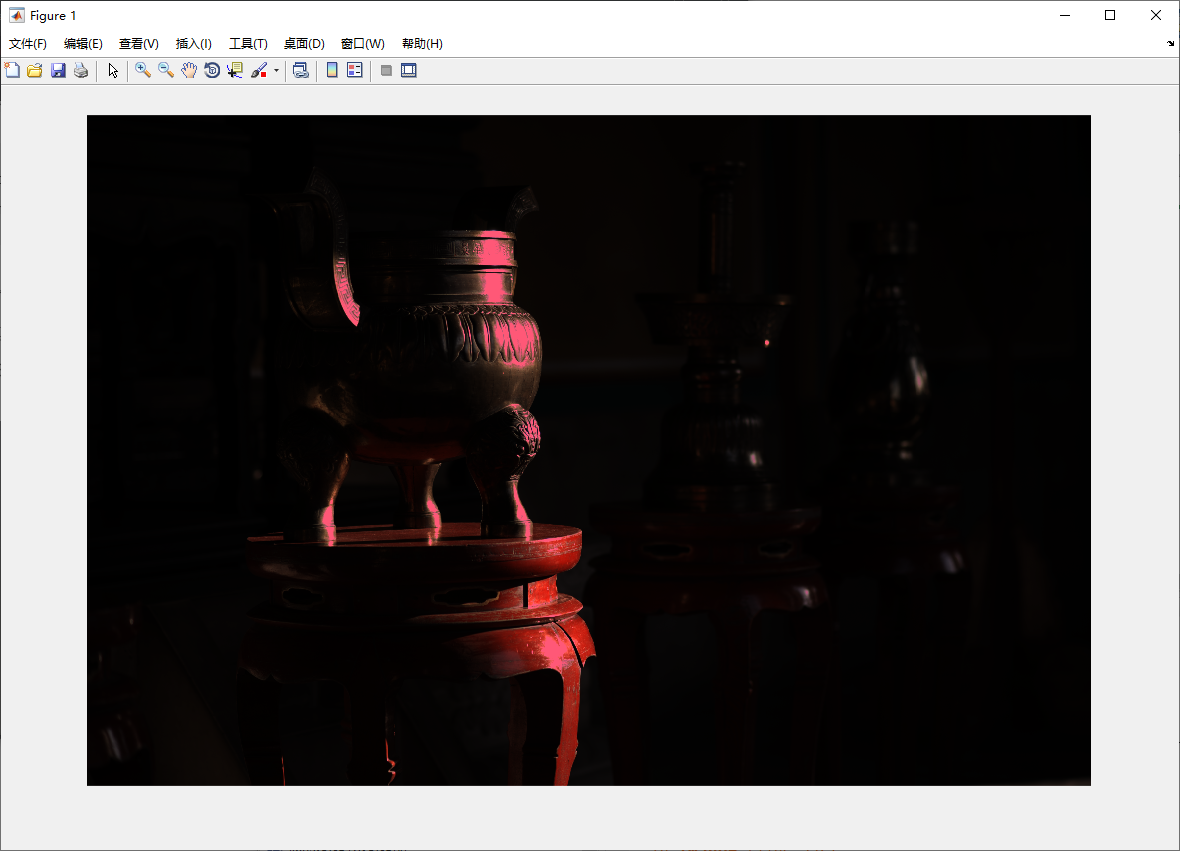◎ 色彩插值后的图像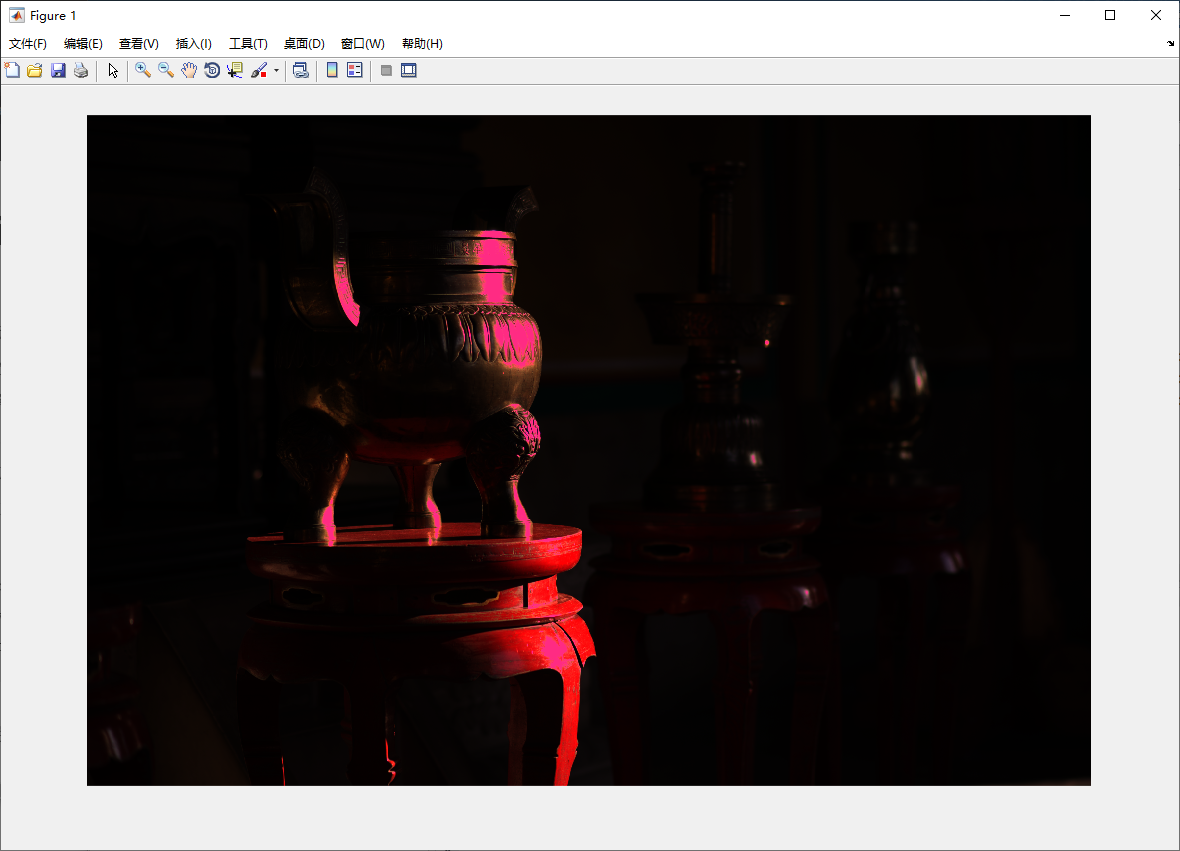◎ 转换至 sRGB 空间后的图像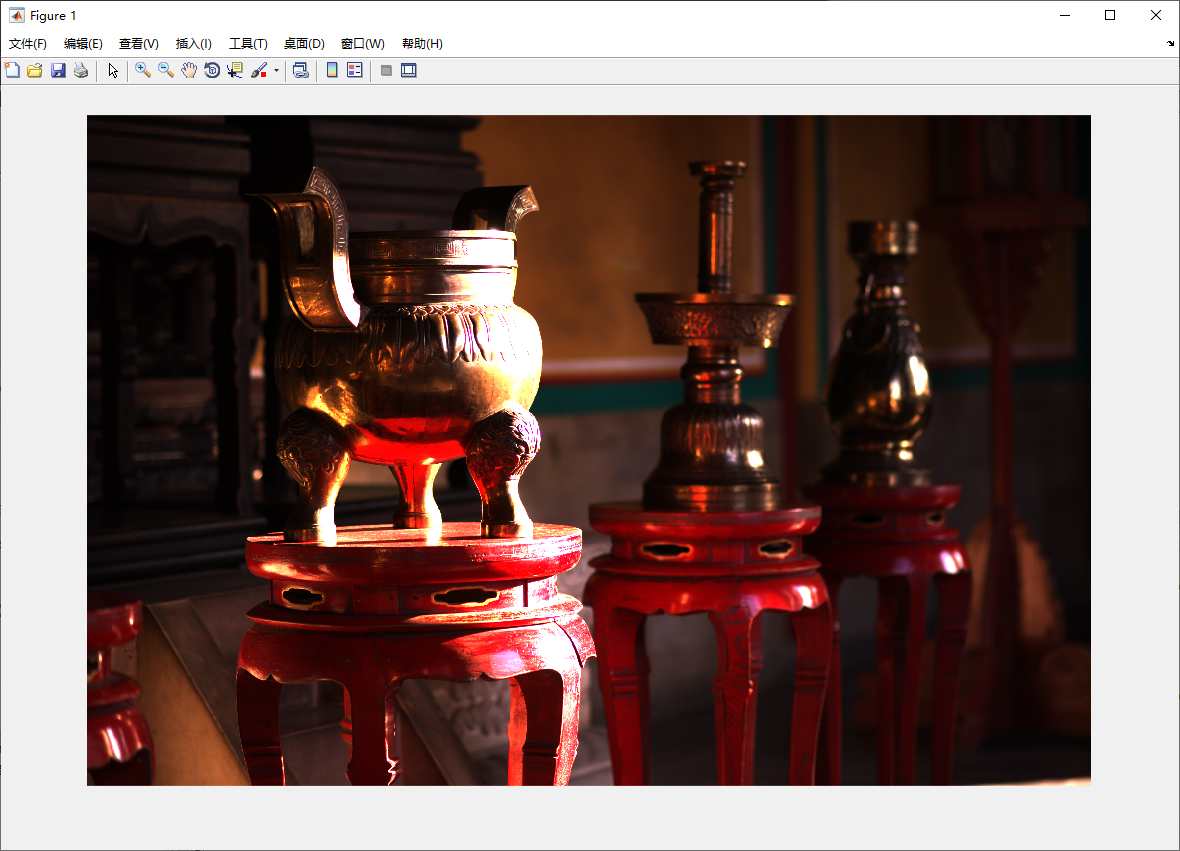◎ 经过亮度校正后的图像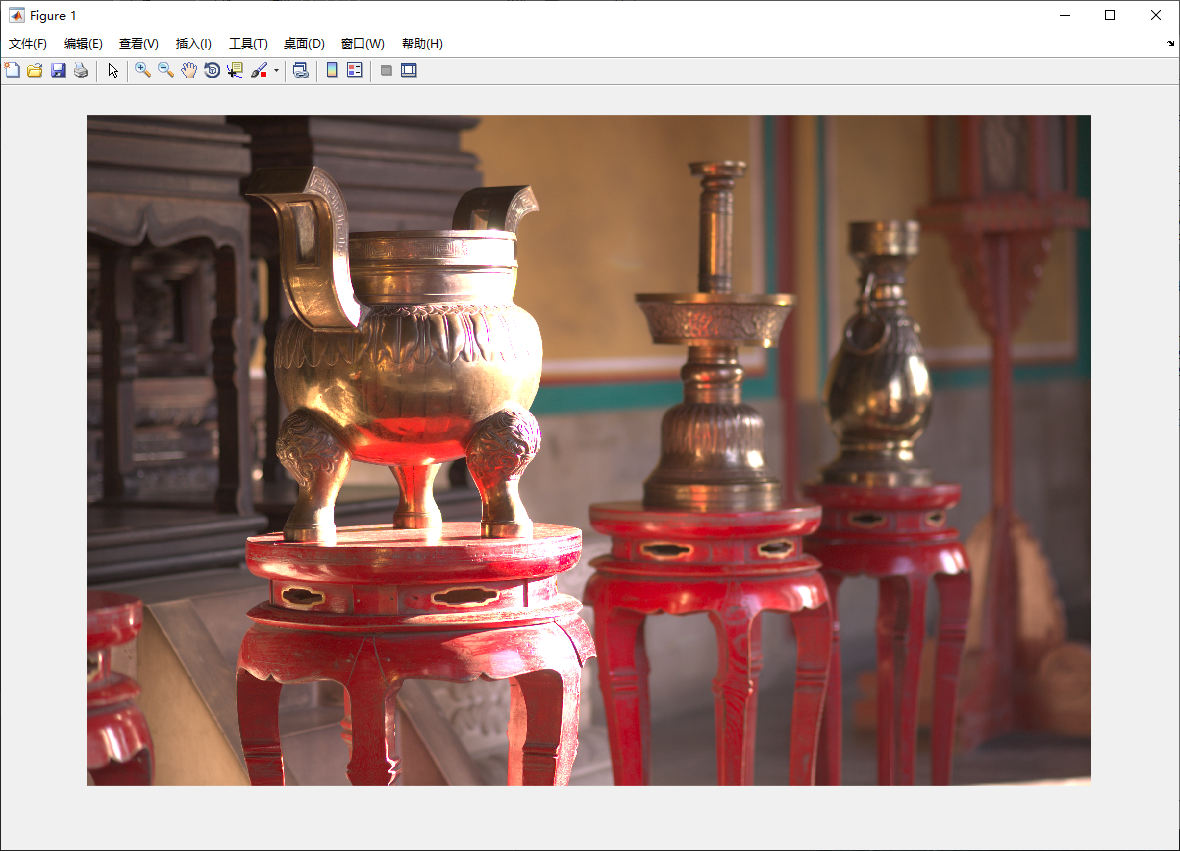◎ 经过 Gamma 校正后的图像

1. 参考：维基百科 | RAW

2. 这里附上 Dcraw 的 C 源代码，为了避免因缺少部分函数库而无法编译，压缩包里也包含了 Windows 系统下的可执行文件。